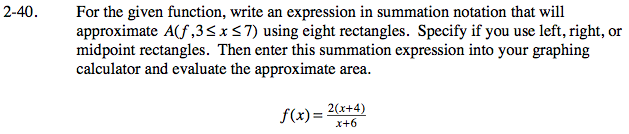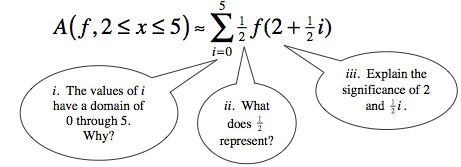### Home > CALC > Chapter Ch2 > Lesson 2.1.3 > Problem2-40

2-40.Recall problem 2-17:i. For left-endpoint and midpoint rectangles, let the index start at 0 and end at n-1.
For right-endpoint rectangles, let the index start at 1 and end at n.
n = number of rectangles.

$\text{ii. The }\frac{1}{2}\text{ represents the WIDTH/BASE of all rectangles.}$

To determine the width of each rectangle, divide the domain by the number of rectangles:

$\frac{7-3}{1}=\frac{1}{2}.$

$\text{Each rectangle has a width, }\Delta x \text{ is } \frac{1}{2}.$

iii. The transformed function represents the HEIGHT of each rectangle.
For left- and right-endpoint rectangles, the heights are represented by f(a + Δxi).
For midpoint rectangles, the heights are represented by

$f \left(a+\frac{\Delta x}{2}i\right).$

a = starting value
Δx = width of each rectangle.